# Split a text column into two columns in Pandas DataFrame

• Difficulty Level : Medium
• Last Updated : 26 Dec, 2018

Let’s see how to split a text column into two columns in Pandas DataFrame.

Method #1 : Using `Series.str.split()` functions.

Split Name column into two different columns. By default splitting is done on the basis of single space by `str.split()` function.

 `# import Pandas as pd ` `import` `pandas as pd ` `  `  `# create a new data frame ` `df ``=` `pd.DataFrame({``'Name'``: [``'John Larter'``, ``'Robert Junior'``, ``'Jonny Depp'``], ` `                   ``'Age'``:[``32``, ``34``, ``36``]}) ` `  `  `print``(``"Given Dataframe is :\n"``,df) ` `  `  `# bydefault splitting is done on the basis of single space. ` `print``(``"\nSplitting 'Name' column into two different columns :\n"``, ` `                                  ``df.Name.``str``.split(expand``=``True``)) `

Output :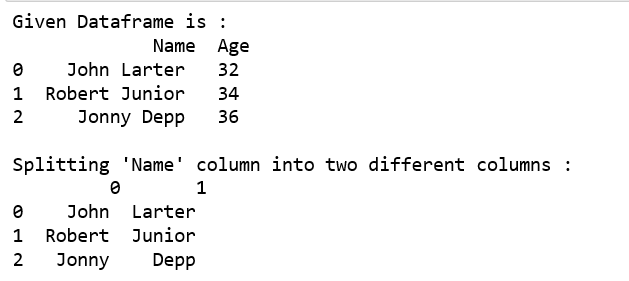Split Name column into “First” and “Last” column respectively and add it to the existing Dataframe .

 `# import Pandas as pd ` `import` `pandas as pd ` `  `  `# create a new data frame ` `df ``=` `pd.DataFrame({``'Name'``: [``'John Larter'``, ``'Robert Junior'``, ``'Jonny Depp'``], ` `                    ``'Age'``:[``32``, ``34``, ``36``]}) ` `  `  `print``(``"Given Dataframe is :\n"``,df) ` `  `  `# Adding two new columns to the existing dataframe. ` `# bydefault splitting is done on the basis of single space. ` `df[[``'First'``,``'Last'``]] ``=` `df.Name.``str``.split(expand``=``True``) ` `  `  `print``(``"\n After adding two new columns : \n"``, df) `

Output: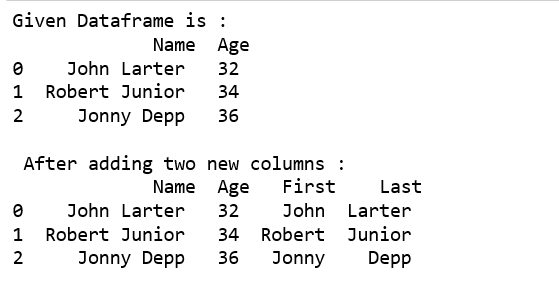Use underscore as delimiter to split the column into two columns.

 `# import Pandas as pd ` `import` `pandas as pd ` `  `  `# create a new data frame ` `df ``=` `pd.DataFrame({``'Name'``: [``'John_Larter'``, ``'Robert_Junior'``, ``'Jonny_Depp'``], ` `                    ``'Age'``:[``32``, ``34``, ``36``]}) ` `  `  `print``(``"Given Dataframe is :\n"``,df) ` `  `  `# Adding two new columns to the existing dataframe. ` `# splitting is done on the basis of underscore. ` `df[[``'First'``,``'Last'``]] ``=` `df.Name.``str``.split(``"_"``,expand``=``True``) ` `  `  `print``(``"\n After adding two new columns : \n"``,df) `

Output :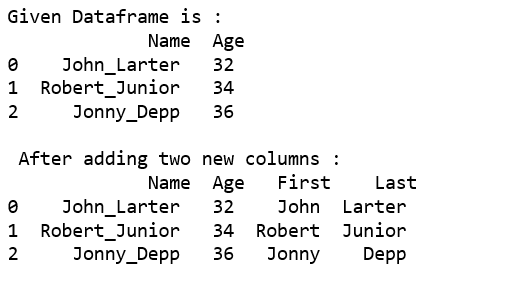Use `str.split()`, `tolist()` function together.

 `# import Pandas as pd ` `import` `pandas as pd ` `  `  `# create a new data frame ` `df ``=` `pd.DataFrame({``'Name'``: [``'John_Larter'``, ``'Robert_Junior'``, ``'Jonny_Depp'``], ` `                    ``'Age'``:[``32``, ``34``, ``36``]}) ` `  `  `print``(``"Given Dataframe is :\n"``,df) ` ` `  `print``(``"\nSplitting Name column into two different columns :"``)  ` `print``(pd.DataFrame(df.Name.``str``.split(``'_'``,``1``).tolist(), ` `                         ``columns ``=` `[``'first'``,``'Last'``])) `

Output :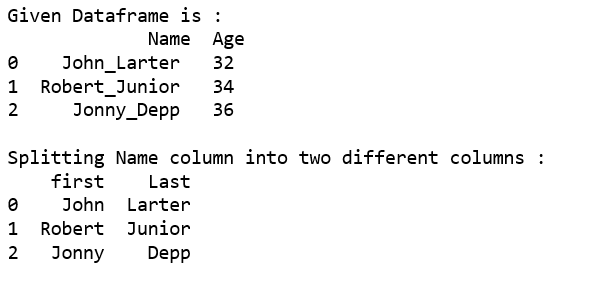Method #2 : Using `apply()` function.

Split Name column into two different columns.

 `# import Pandas as pd ` `import` `pandas as pd ` `  `  `# create a new data frame ` `df ``=` `pd.DataFrame({``'Name'``: [``'John_Larter'``, ``'Robert_Junior'``, ``'Jonny_Depp'``], ` `                    ``'Age'``:[``32``, ``34``, ``36``]}) ` `  `  `print``(``"Given Dataframe is :\n"``,df) ` ` `  `print``(``"\nSplitting Name column into two different columns :"``)  ` `print``(df.Name.``apply``(``lambda` `x: pd.Series(``str``(x).split(``"_"``)))) `

Output :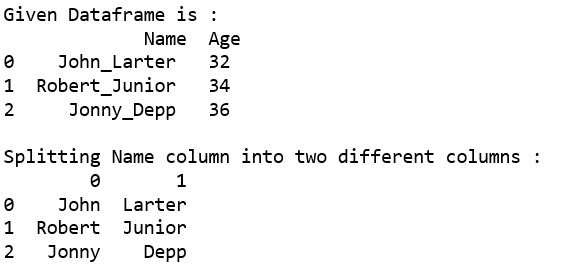Split Name column into two different columns named as “First” and “Last” respectively and then add it to the existing Dataframe.

 `# import Pandas as pd ` `import` `pandas as pd ` `  `  `# create a new data frame ` `df ``=` `pd.DataFrame({``'Name'``: [``'John_Larter'``, ``'Robert_Junior'``, ``'Jonny_Depp'``], ` `                    ``'Age'``:[``32``, ``34``, ``36``]}) ` `  `  `print``(``"Given Dataframe is :\n"``,df) ` ` `  `print``(``"\nSplitting Name column into two different columns :"``)  ` ` `  `# splitting 'Name' column into Two columns  ` `# i.e. 'First' and 'Last'respectively and  ` `# Adding these columns to the existing dataframe. ` `df[[``'First'``,``'Last'``]] ``=` `df.Name.``apply``( ` `   ``lambda` `x: pd.Series(``str``(x).split(``"_"``))) ` `  `  `print``(df) `

Output :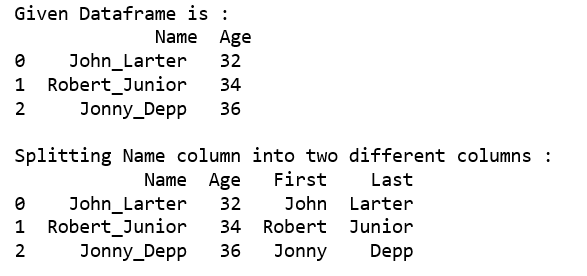My Personal Notes arrow_drop_up
Recommended Articles
Page :